N.B. A detailed on-line essay by S. Finch was the starting point for this entry.

Letbe the number of ways in which the Factorial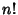can be decomposed intoFactors of the form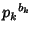arranged in nondecreasing order. Also define(1)

i.e.,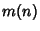is the Least Prime Factor raised to its appropriate Power in the factorization. Then define(2)

where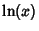is the Natural Logarithm. For instance,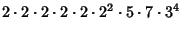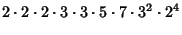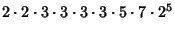(3)

so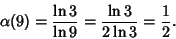(4)

For large,(5)

where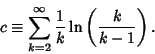(6)

References

Alladi, K. and Grinstead, C. On the Decomposition ofinto Prime Powers.'' J. Number Th. 9, 452-458, 1977.

Finch, S. Favorite Mathematical Constants.'' http://www.mathsoft.com/asolve/constant/aldgrns/aldgrns.html

Guy, R. K. Factorialas the Product ofLarge Factors.'' §B22 in Unsolved Problems in Number Theory, 2nd ed. New York: Springer-Verlag, p. 79, 1994.